Function Repository Resource:

# IndexedOrderedTuple

Give the index of an ordered tuple or return the ordered tuple with that index

Contributed by: Ed Pegg Jr
 ResourceFunction["IndexedOrderedTuple"][choose,index] returns an ordered tuple of length choose with given index. ResourceFunction["IndexedOrderedTuple"][list] gives the index of ordered tuple list.

## Details and Options

For any positive integers n and k, n can be uniquely represented as k binomials,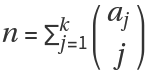, where the aj terms are strictly increasing.

## Examples

### Basic Examples (3)

Generate a list of the first ten 3-tuples:

 In:=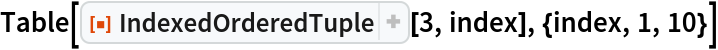Out=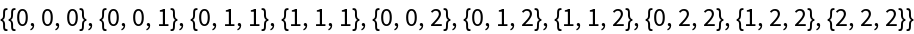The function can return ordered tuples of a large index:

 In:=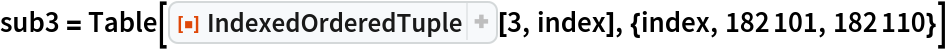Out=Applying the function to an ordered tuple returns the index:

 In:=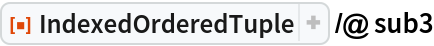Out=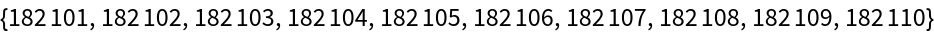Any increasing list of integers can be considered as a ordered tuple with a unique index:

 In:=Out=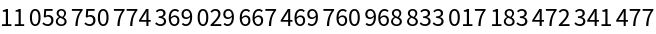The index above generates a unique ordered 10-tuple:

 In:=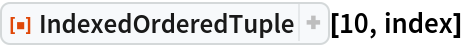Out=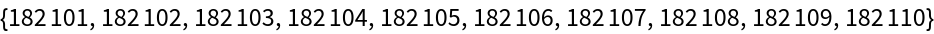### Scope (3)

Here are some ordered 2-tuples with their indices to show their structure:

 In:=Out=The ordered 2-tuple {4,4} has an index of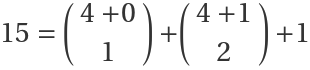:

 In:=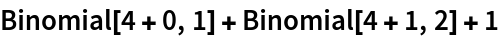Out=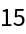Recover the same information with IndexedOrderedTuple:

 In:=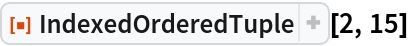Out=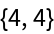The structure of ordered 3-tuples in 3D:

 In:=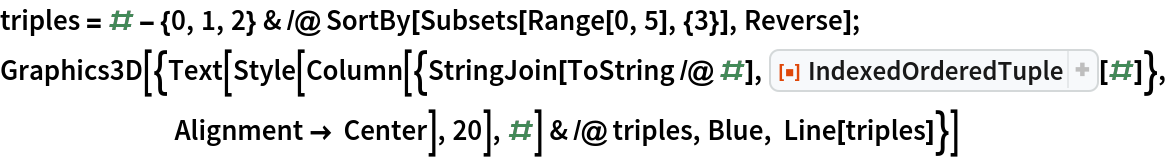Out=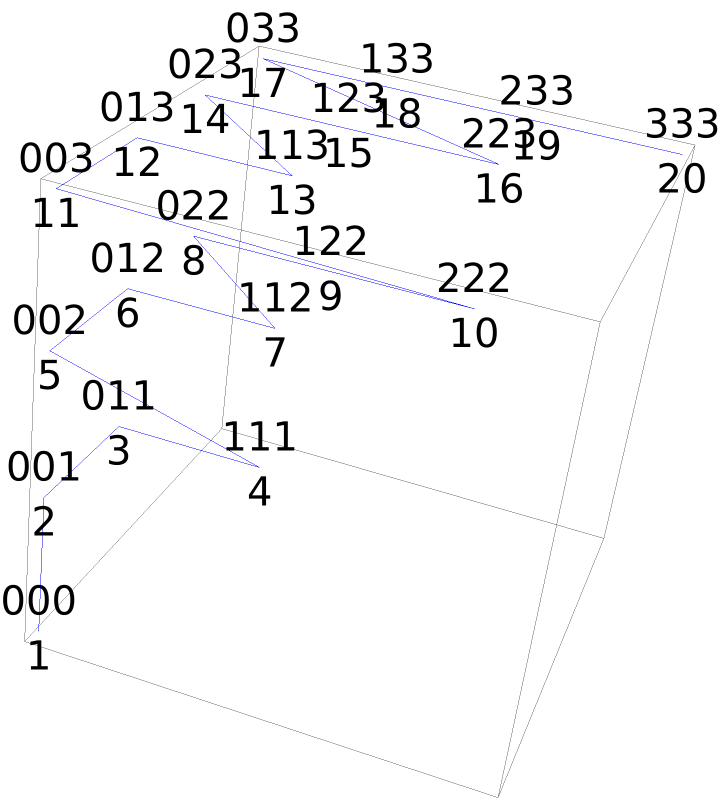### Properties and Relations (3)

Use Tuples to produce ordered 3-tuples:

 In:=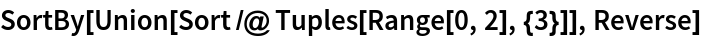Out=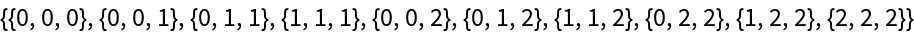The same sequence of ordered 3-tuples can be obtained from ordered Subsets:

 In:=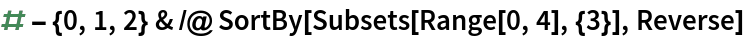Out=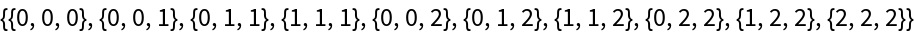IndexedOrderedTuple returns the same ordered tuples in the same sequence:

 In:=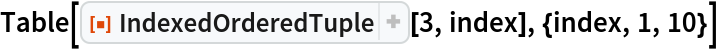Out=## Version History

• 1.0.0 – 07 November 2019